Analog Electronics - 7

# Analog Electronics - 7 - Electrical Engineering (EE)

Test Description

## 10 Questions MCQ Test Analog Electronics - Analog Electronics - 7

Analog Electronics - 7 for Electrical Engineering (EE) 2023 is part of Analog Electronics preparation. The Analog Electronics - 7 questions and answers have been prepared according to the Electrical Engineering (EE) exam syllabus.The Analog Electronics - 7 MCQs are made for Electrical Engineering (EE) 2023 Exam. Find important definitions, questions, notes, meanings, examples, exercises, MCQs and online tests for Analog Electronics - 7 below.
Solutions of Analog Electronics - 7 questions in English are available as part of our Analog Electronics for Electrical Engineering (EE) & Analog Electronics - 7 solutions in Hindi for Analog Electronics course. Download more important topics, notes, lectures and mock test series for Electrical Engineering (EE) Exam by signing up for free. Attempt Analog Electronics - 7 | 10 questions in 30 minutes | Mock test for Electrical Engineering (EE) preparation | Free important questions MCQ to study Analog Electronics for Electrical Engineering (EE) Exam | Download free PDF with solutions
 1 Crore+ students have signed up on EduRev. Have you?
Analog Electronics - 7 - Question 1

### For the Wien-bridge oscillator circuit shown below, the condition for bridge balance is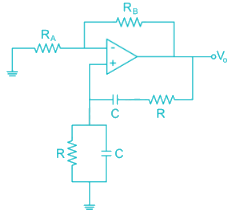Detailed Solution for Analog Electronics - 7 - Question 1

At oscillator frequency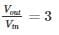i.e. gain of non-inverting amplifier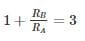⇒ RB = 2RA

Analog Electronics - 7 - Question 2

### Find the value of inductance in (mH) in the oscillator shown below to achieve a frequency of oscillation = 100 KHz is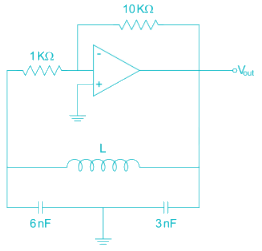Detailed Solution for Analog Electronics - 7 - Question 2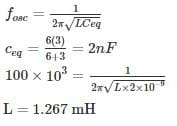*Answer can only contain numeric values
Analog Electronics - 7 - Question 3

### For the circuit shown in the figure the value of R to get oscillation of 100 kHz is ________ KΩ.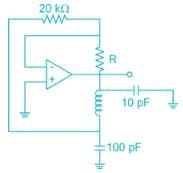Detailed Solution for Analog Electronics - 7 - Question 3

The given oscillator is Colpitts’s oscillator

The condition for oscillation is

|Aβ|=1

A = -R2/R1

β=C2/C1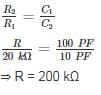*Answer can only contain numeric values
Analog Electronics - 7 - Question 4

The figure shows a phase-shift oscillator circuit with voltage follower buffer stages.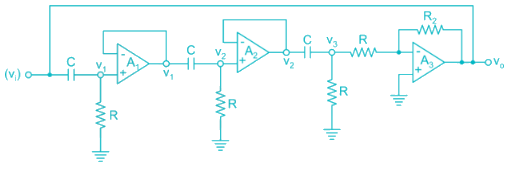The value of R2/R required for sustained oscillations is _____.

Detailed Solution for Analog Electronics - 7 - Question 4
• The basic amplifier of the circuit is the op-amp A3.
• The output of amplifier is connected to 3 stage RC filter.

Transfer function of first RC network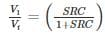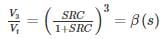The amplifier A3 is connected in inverting mode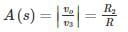The loop gain is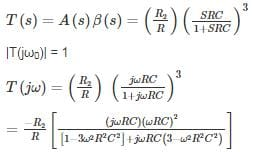Substitute real part of denominator to zero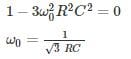At this frequency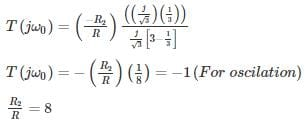Analog Electronics - 7 - Question 5

The value of R in oscillator circuit shown in a given circuit below is chosen such that it oscillates at an angular frequency of ω. The value of ω and the required value of R will respectively be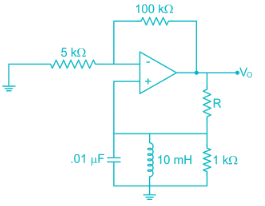Detailed Solution for Analog Electronics - 7 - Question 5

Hartley oscillator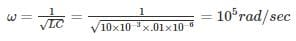Amp with +ve F.B

Loop again AB = 1 + j0

At oscillation

∠AB = 0 (no reactance XL = XC)

At frequency of oscillation look lies resistive Network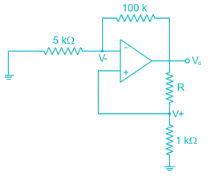For ideal op amp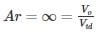Use voltage division Rule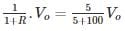R + 1 = 21

R = 20 kΩ

R = 2 × 104Ω

*Answer can only contain numeric values
Analog Electronics - 7 - Question 6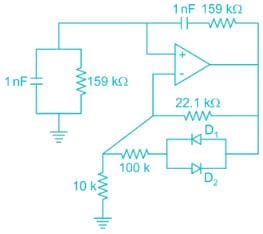The frequency of oscillation of the circuit in kilohertz is ______ kHz.

Detailed Solution for Analog Electronics - 7 - Question 6

The given circuit is wein bridge oscillator.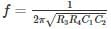R3 = R4 = 159 KΩ

C1 = C2 = 1 nF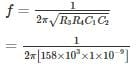= 103 HZ

= 1 KHZ

Analog Electronics - 7 - Question 7

For the transistor oscillator circuit given below the frequency of oscillation is 8.5 kHz. The value of the collector resistor approximately is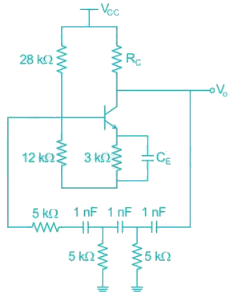Detailed Solution for Analog Electronics - 7 - Question 7

In a transistor phase shift oscillator the oscillation frequency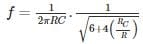R, C are the resistor and the capacitor in phase shift circuit

RC is collector capacitance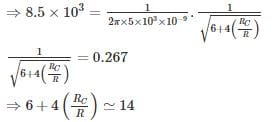⇒ RC = 2R = 10 kΩ

*Answer can only contain numeric values
Analog Electronics - 7 - Question 8

The oscillation frequency if the below-shown oscillator is 1 kHz then the value of ‘L’ is ______ mH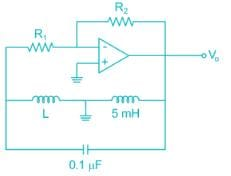Detailed Solution for Analog Electronics - 7 - Question 8

The given oscillator is Hartley oscillator, where the oscillation frequency.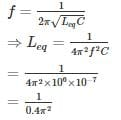= 253.3 mH

Leq = L + 5 mA

⇒ L = 253.3 – 5

= 248. 3 mH

Analog Electronics - 7 - Question 9

For the circuit shown the value of R1 and R2 such that the circuit generates oscillations of frequency 274 Hz is [Assume the time constant of the feedback RC network is 0.01 sec]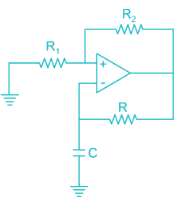Detailed Solution for Analog Electronics - 7 - Question 9

Given figure is of A-stable multi-vibrator,

Time constant is given by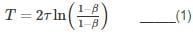β = feedback factor

τ = time constant of feedback loop: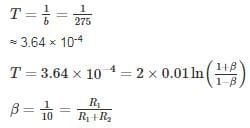Option 3 satisfies the condition

*Answer can only contain numeric values
Analog Electronics - 7 - Question 10

The frequency of oscillation of the circuit shown below is ________ Hz.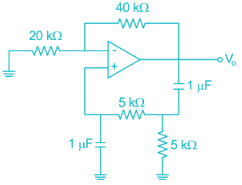Detailed Solution for Analog Electronics - 7 - Question 10

The given oscillator is Wien-bridge oscillator with R = 5 kΩ, C = 1 μF

⇒ frequency of oscillation f = 1/2πRC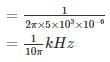= 31.8 Hz

## Analog Electronics

11 videos|57 docs|108 tests
Information about Analog Electronics - 7 Page
In this test you can find the Exam questions for Analog Electronics - 7 solved & explained in the simplest way possible. Besides giving Questions and answers for Analog Electronics - 7, EduRev gives you an ample number of Online tests for practice

## Analog Electronics

11 videos|57 docs|108 tests(Scan QR code)